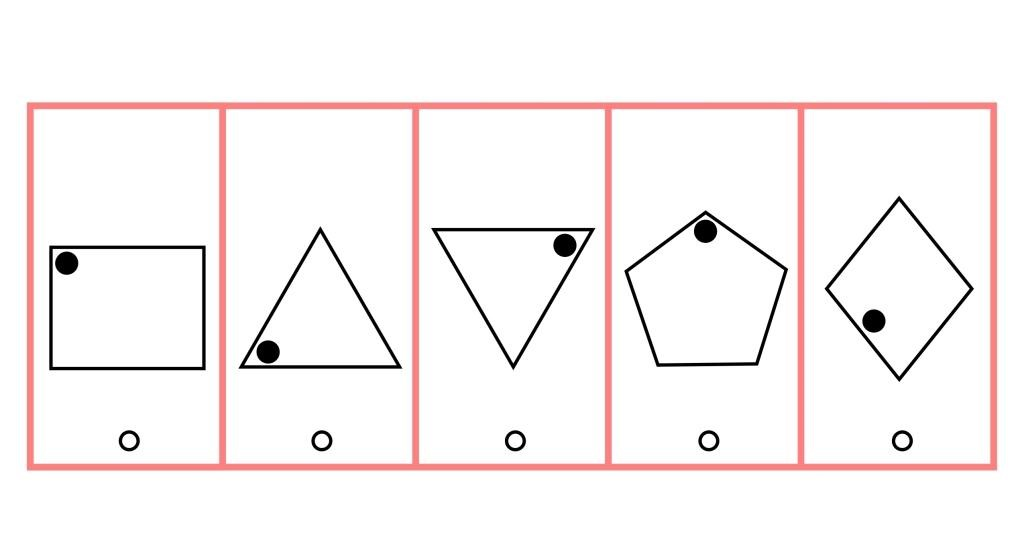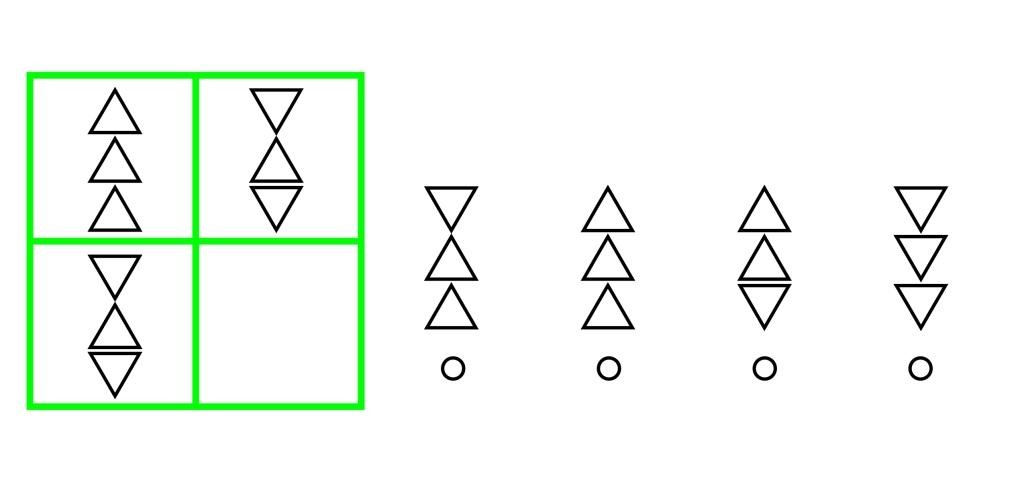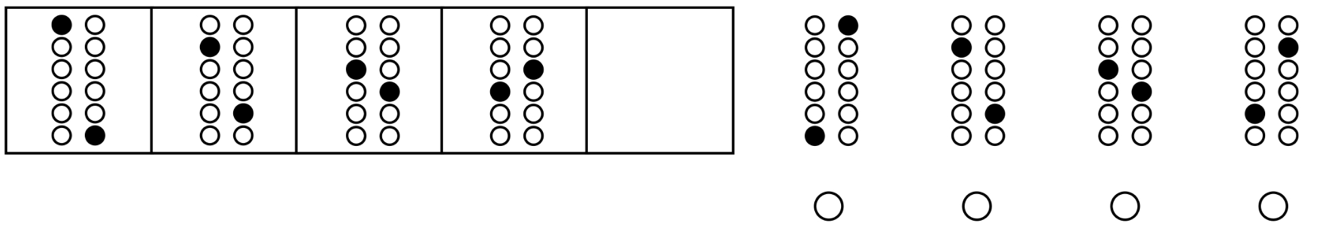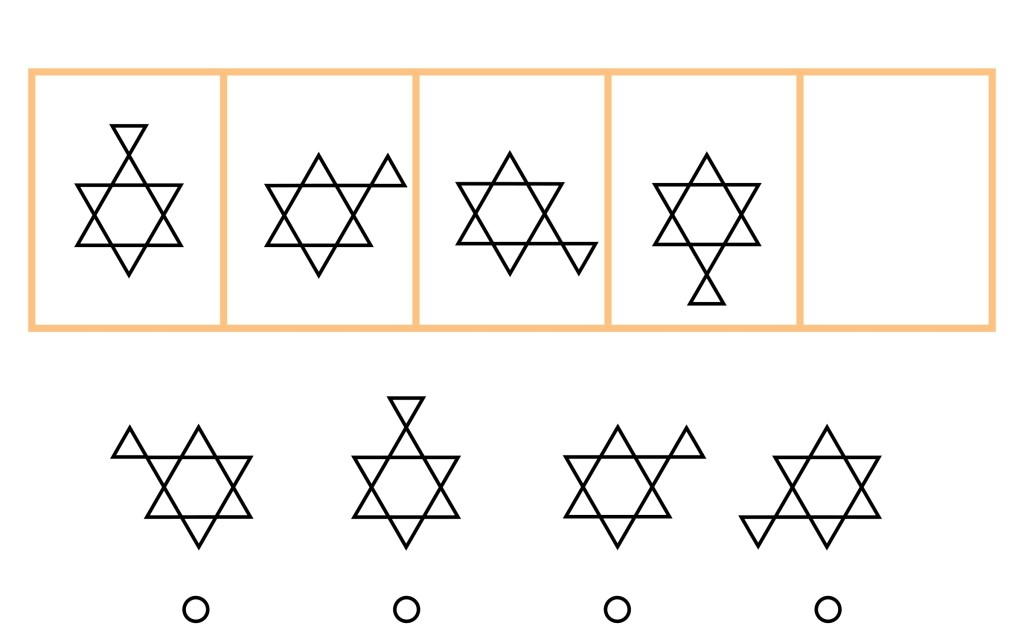Start Now with 100 Free Questions

# OLSAT Figural Reasoning

This section of the OLSAT test measures the comprehension of the relationship between various geometrical shapes and figures, as well as patterns and progressions, and how they reason their way through non-language based scenarios. Much like pictorial reasoning, students are expected to find the relationship between elements and/or objects in a pattern, and are asked to predict and create what the next level of the pattern will look like, thereby generalizing the rules they discover. This section assesses a child’s ability to determine relationships with numbers as well as figure out and utilize computational rules. Figural Reasoning consists of figural classification, figural analogy, pattern matrix and figural series.

## Figural Classification

Students must examine a group of figures and identify a pattern or principle that links those figures. Then, students must determine which of the answer choices follows this same principle. Figural Classification is on levels A-D of the OLSAT, which covers Kindergarten through 3rd grade.

### Sample Question:

Look at the figures in the boxes. One of the figures in the boxes does not belong with the others because it is different in some way.  Can you point to the picture that doesn’t belong?5 – the dot isn’t in a corner – Level A

## Figural Analogy

With these questions, students are asked to examine the relationship between figures. Once a relationship rule is concluded, students must apply this rule to a second pair of figures. The student’s must infer a relationship between a pair of geometric shapes and then select the shape that is related to the stimulus in the same way. Figural Analogy is on levels A-G of the OLSAT, which covers Kindergarten through 12th grade.

### Sample Question:

Look at the shapes in the top two boxes.  Do you see how they go together in a certain way?  Now look at the shapes on the side.  Which shapes go with the shapes on the bottom row the same way that the shapes in the top row go together?2 – the top and bottom triangles flip over – Level C

## Pattern Matrix

The student is asked to supply a missing element in a matrix of geometric shapes in these questions. This tests the student’s ability to discern rules and evaluate how those rules govern a series of geometric figures as they run horizontally and vertically across the matrix. Pattern Matrix is on levels A-G of the OLSAT, which covers Kindergarten through 12th grade.

### Sample Question:

What belongs in the empty box to complete the pattern?## Figural Series

Students must look at a series of geometric figures, and then discern a pattern within the series. After a pattern is determined, they then predict the ‘next’ drawing/shape in the pattern. Figural Series is on levels A-G of the OLSAT, which covers Kindergarten through 12th grade.

### Sample Question:

What belongs in the empty box to complete the pattern?4 – the triangle is rotating around the star in a clockwise direction – Level B